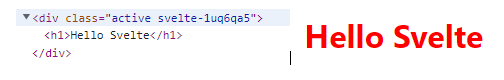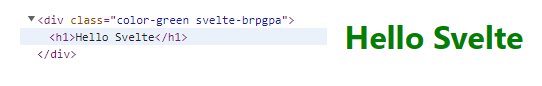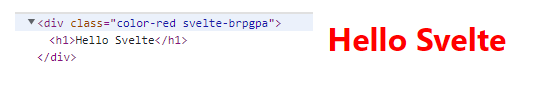# How to conditionally bind class in Svelte

## 📋 Table Of Content

In this post, we will learn how to bind classes in Svelte Js.

Let's first create a class name active and give it a style of color: red;

Now we can use it in our div like this.

``````<div class="active">Hello World</div>
``````

This is an example of a static class, and this will change the text color to red.

However, in Svelte we can also add the same class in a dynamic way using the `{}` brackets. This helps to bind a javascript expression to our class attribute.

These dynamic classes helps to add or remove the classes depending on changes.

``````<script>
let myActiveClass = 'active'
</script>

<main>
<div class={myActiveClass}>
<h1>Hello Svelte</h1>
</div>
</main>
``````

Here, we have assigned the active class to a variable myActiveClass in the script tag. And the curly braces help to bind the variable to the class attributes.

The output will be the same, it will change the color of the text to red.Next, let's see how we can bind classes depending on conditions in svelte.

## Conditional binding of classes in Svelte

Let's first create two classes color-red and color-green.

``````.color-red{
color:red;
}
.color-green{
color: green
}
``````

And a boolean variable in the script tag.

``````<script>
let isTrue = false;
</script>
``````

We have created a boolean variable isTrue and initially set it to `false`.

Next, we will do class binding and inside the curly braces `{}` we will use the ternary operator to conditionally changes our classes.

``````<script>
let isTrue = false;
</script>

<main>
<div class={isTrue ? 'color-red' : 'color-green'}>
<h1>Hello Svelte</h1>
</div>
</main>
``````

Here, if isTrue is `true` , it will apply the 'color-red' class or else apply the 'color-green' class.

Since, in the example, the isTrue is `false` the color-green class is applied to the text.If isTrue is set to `true`, the color-red class is applied to the text.Now since conditionally changing classes is very common while working with the frameworks, that svelte have special directives to do this same work more easily.

For example, we can apply the color-red class when isTrue is set to `true` like this.

``````<script>
let isTrue = true;
</script>

<main>
<div class:color-red={isTrue}>
<h1>Hello Svelte</h1>
</div>
</main>
``````

Or, if the variable name and class name is the same we can just use the shorthand.

``````<script>
let color-red = true;
</script>

<main>
<div class:color-red>
<h1>Hello Svelte</h1>
</div>
</main>

<style>
.color-red {
color: red;
}
</style>
``````

Related Articles:

How to bind text, html and attributes in Svelte